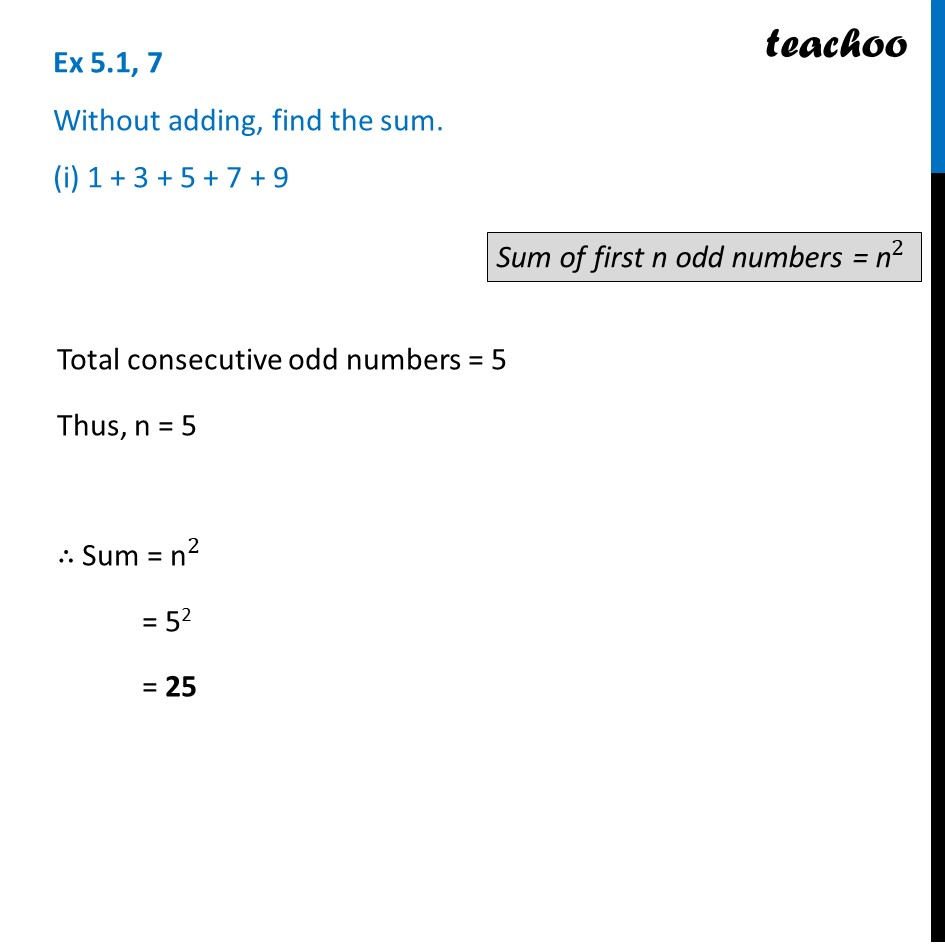Sum of consecutive odd numbers

Chapter 5 Class 8 Squares and Square Roots
Concept wiseLearn in your speed, with individual attention - Teachoo Maths 1-on-1 Class

### Transcript

Ex 5.1, 7 Without adding, find the sum. (i) 1 + 3 + 5 + 7 + 9 Total consecutive odd numbers = 5 Thus, n = 5 ∴ Sum = "n" ^2 = 52 = 25 Sum of first n odd numbers = "n" ^2